Robert Cowen

## Mixing Numbers and Unfriendly Colorings of GraphsNBPDF

Mathematica has many built-in functions for doing research in graph theory. Formerly it was necessary to load the Combinatorica package to access these functions; most are now available within Mathematica itself. This article studies a problem concerning the vertex coloring of graphs using Mathematica by introducing some user-defined functions.

### Unfriendly Colorings of Graphs

We only consider vertex colorings, so a “colored graph” always means a vertex-colored graph. An-coloring of a graph is a partition of the vertices intodisjoint subsets. We start with 2-colorings; call the colors red and blue. Two vertices are neighbors if they are connected by an edge. We say that two vertices of the same color are friends and two vertices of opposite colors are strangers. If more than half the neighbors of a colored vertexare friends of, we say thatlives in a friendly neighborhood; otherwise,is said to live in an unfriendly neighborhood. If all the vertices of the graph have the same color, every vertex lives in a friendly neighborhood. Is there a 2-coloring such that every vertex lives in an unfriendly neighborhood? The surprising answer to this question is yes, as we shall show.

A 2-coloring of a graph is unfriendly if each vertex lives in an unfriendly neighborhood, that is, at least half its neighbors are colored differently from itself. It is a theorem that every finite graph has an unfriendly coloring. (The situation is much more complicated for infinite graphs [1, 2]). The proof is clever, but not very long and we give it next. Define the mixing number of a colored graph to be the number of its edges whose vertices have different colors. Proceed by successively “flipping,” that is, changing the color of those vertices that live in friendly neighborhoods. When a vertex is flipped, it may change the neighborhood status of other vertices; however, each flip increases the mixing number of the graph. Since the mixing number is bounded by the number of edges in the graph, this flipping process must eventually end with no more flippable vertices, that is, no more vertices living in friendly neighborhoods.

### Finding the Mixing Number of a Colored Graph

We start with an easy example. We first consider the complete graph with 7 vertices.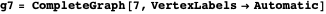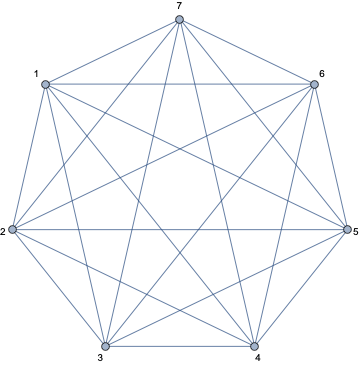We will color the vertices either red or blue. Assume that we start with four blue and three red vertices.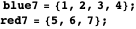Here is how we show the colors.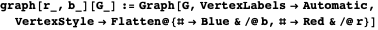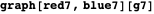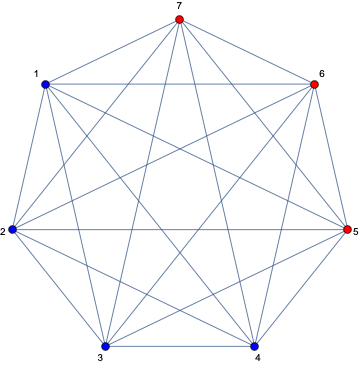To calculate the mixing number, we first generate the set of edges.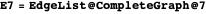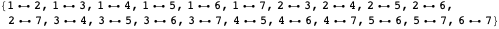Next we introduce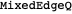, which determines if the vertices of an edge have the different colors.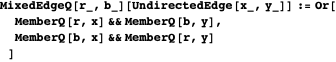Then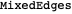selects those edges whose vertices have different colors.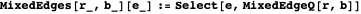Finally, we applyto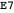.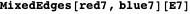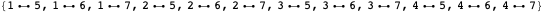This is the mixing number of.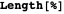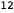It is easy to see by considering the other 2-colorings of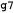that this coloring has the maximum mixing number for the graph and hence this coloring must be unfriendly. Four of one color and three of the other gives a mixing number of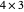, whereas five of one color and two of the other gives a mixing number of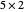, and so on. Of course, a direct inspection also shows the coloring is unfriendly.

### A Larger Example

We used the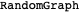function to construct a graph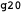with 20 vertices and 100 edges. Here is the list of edges that was generated.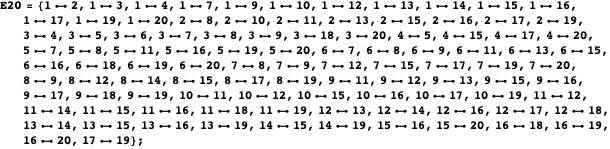Arbitrarily color the first 10 vertices red and the remaining 10 blue.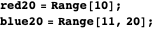Here is the image of the graph, colored as before.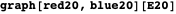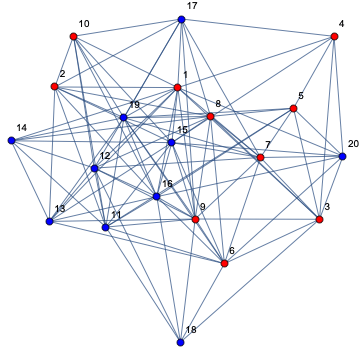The function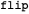changes the color of a vertex.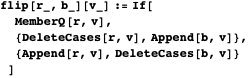For example, this flips vertex 1.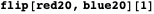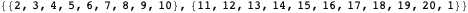We need to get the set of neighbors of a vertexin a graph, that is, those vertices that share an edge with. Since the built-in Mathematica function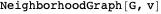includes the vertexitself, we remove it.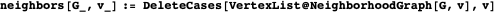For example, here are the neighbors of vertex 3 in.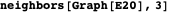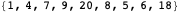Next we obtain the edges whose vertices are colored differently.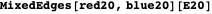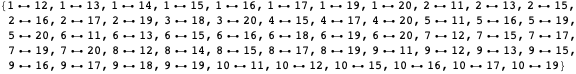The length of that list is the mixing number of the graph with edges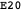and the color partition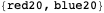.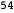Call a vertexa secure vertex in a graphiflives in a friendly neighborhood, that is,has the same color as most of its neighbors in.

The function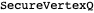determines whetheris a secure vertex inwith the color partition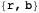.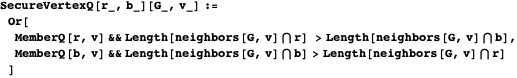For example, these are the secure vertices of.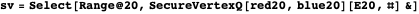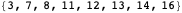We define the corresponding function,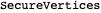.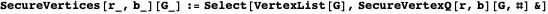### One Step of Unfriendly Coloring a Graph

We select the first elementin the list of secure vertices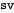and set the colors.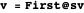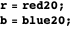We flip the color ofsince it lives in a friendly neighborhood, or equivalently, it is a secure vertex.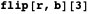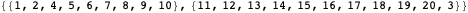We could now repeat this step using the generated color partition and stop when there are no more secure vertices. In the next section we write the function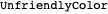to carry out the process to the end, with output the final color partition.

### Unfriendly Coloring a Graph

The following short program produces an unfriendly coloring of any 2-colored graph starting with the color partition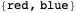.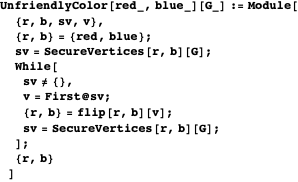Here is the unfriendly color partition.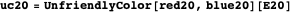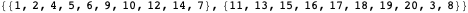Here is the unfriendly coloring of the graph.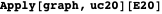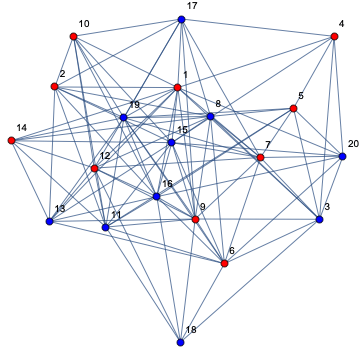We can even start with all vertices having the same color, say red.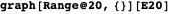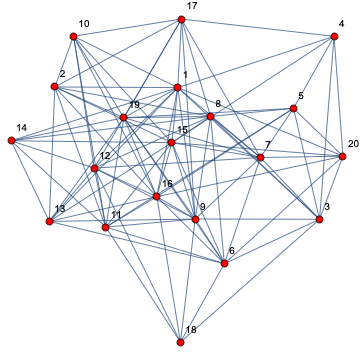We run our program and use the new color partition.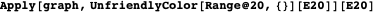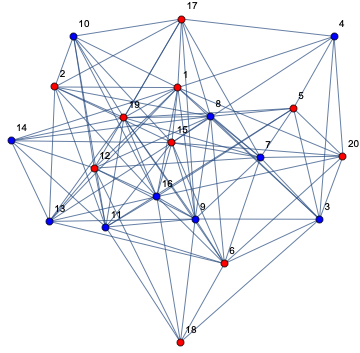### Relation to the Max-Cut Problem

The max-cut problem is to partition the vertices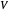of a graph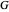into two setsand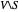so as to maximize the number of edges whose endpoints are in both sets. However, this is equivalent to a two-coloring of the vertices ofsuch that the size of the max cut (i.e. the number of edges joining the two cut sets) is the same as the mixing number of the coloring. The max-cut problem is known to be NP-hard [3, 4], which implies that methods of finding this maximum will not run in polynomial time unless P = NP, something most mathematicians consider unlikely.

In the context of the max-cut problem, our procedure,, is known as local search and can easily be shown to always produce a cut with at least half the number of edges in the graph .

### Conclusion

We have shown how to find unfriendly 2-colorings of finite graphs, which can easily be extended to more colors. We feel that treating a vertex coloring as a partition of the list of vertices and regarding this as an integral and dynamic part of the graph should be of use in investigating other coloring problems.

Finally, I wish to dedicate this work to Bill Emerson, who worked on this problem with me over 30 years ago (see reference 3 in  to our unpublished paper). Bill was a very talented mathematician and a good friend. He is missed by all who knew him.

### Acknowledgements

I am grateful to George Beck for improving my programming throughout the paper and to Professor Lenore Cowen for pointing out the connection to the max-cut problem.

### References

  R. Aharoni, E. C. Milner and K. Prikry, “Unfriendly Partitions of a Graph,” Journal of Combinatorial Theory, Series B, 50(1), 1990 pp. 1–10. https://doi.org/10.1016/0095-8956(90)90092-E.  S. Shelah and E. C. Milner, “Graphs with No Unfriendly Partitions,” A Tribute to Paul Erdős (A. Baker, B. Bollobás and A. Hajnal, eds.), Cambridge, UK: Cambridge University Press, 1990 pp. 373–384.  D. Panigrahi, “COMPSCI 638: Graph Algorithms, Lecture 22.” (Sep 10, 2021) https://courses.cs.duke.edu//fall19/compsci638/fall19_notes/lecture22.pdf.  M. R. Garey, D. S. Johnson and L. Stockmeyer, “Some Simplified NP-Complete Problems,” in Proceedings of the Sixth Annual ACM Symposium on Theory of Computing, New York: ACM, Seattle, WA, 1974 pp. 47–63. https://dl.acm.org/doi/10.1145/800119.803884. R. Cowen, “Mixing Numbers and Unfriendly Colorings of Graphs,” The Mathematica Journal, 2021. doi.org/10.3888/tmj.23–4.